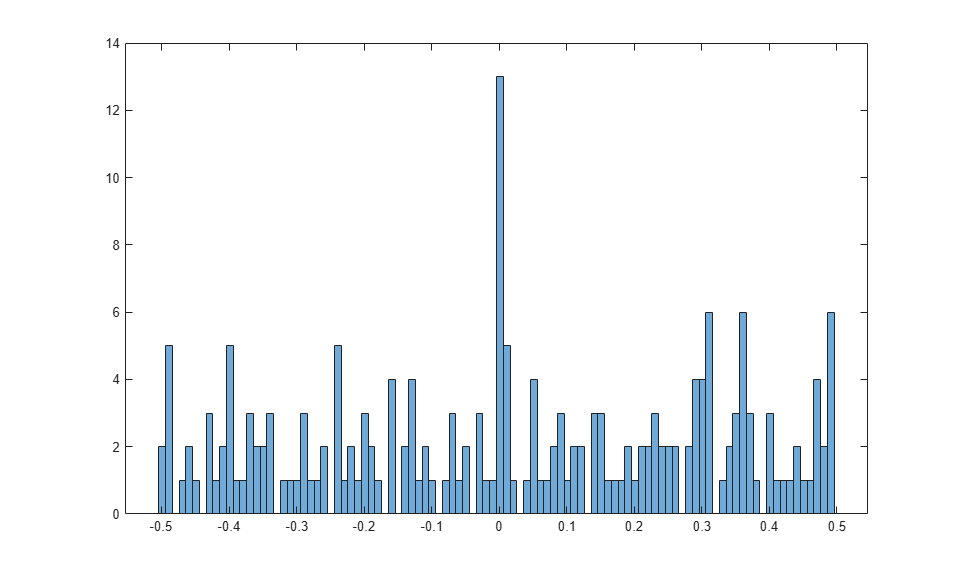Numerical Computations with High Precision

This example shows how to use variable-precision arithmetic to obtain high precision computations using Symbolic Math Toolbox™.

Search for formulas that represent near-integers. For example, compute exp(sqrt(163)*pi) to 30 digits. The result appears to be an integer that is displayed with a rounding error.

disp("Setting precision to 30 digits")
digits(30);
f = exp(sqrt(sym(163))*sym(pi));
vpa(f)
Setting precision to 30 digits

ans =

262537412640768743.999999999999

When you compute the same value to 40 digits, you can see that the value is not actually an integer.

disp("Setting precision to 40 digits")
digits(40);
vpa(f)
Setting precision to 40 digits

ans =

262537412640768743.9999999999992500725972

Next, consider numbers whose values are up to exp(1000). Determine the correct digits after the decimal point to compute these numbers properly. Find the minimum required working precision for the upper bound exp(1000).

disp("Compute the required working precision")
disp(">> d = log10(exp(vpa(1000)))")
d = log10(exp(vpa(1000)))
Compute the required working precision
>> d = log10(exp(vpa(1000)))

d =

434.2944819032518276511289189166050822944

Set the required precision before the first call to a function that depends on it. Using, for example, a function such as round, vpa, or double.

digits(ceil(d) + 50);

Now, consider the numbers of the form exp(sqrt(n)*pi) for n from 1 to 1000. Check if numbers of this form are close to some integer. You can see this from a histogram plot of their fractional parts.

A = exp(pi*sqrt(vpa(1:1000)));
B = A-round(A);
histogram(double(B), 50)Calculate if there are near-integers of the form exp(n).

A = exp(vpa(1:1000));
B = A-round(A);
find(abs(B) < 1/1000);

Now you can see that the fractional parts of the elements of A are rather evenly distributed.

histogram(double(B), 50)